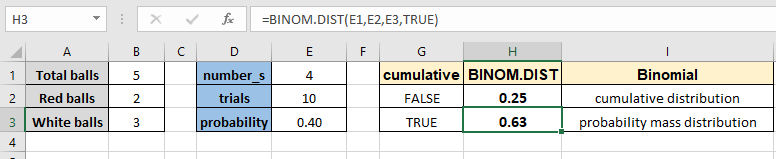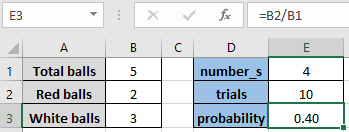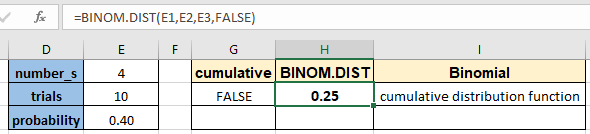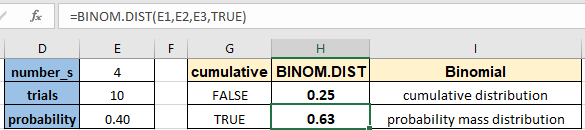# How to use the BINOM.DIST function in ExcelIn this article, we will learn How to use the BINOM.DIST function in Excel.

What is binomial probability distribution and binomial cumulative distribution?

The Binomial Distribution is a statistical measure that is mostly used to find the probability of a specific number of successes occurring from a specific number of independent trials. For example finding the probability of winning an election of a candidate from a sample of votes. binomial distribution gives us an expected probability of the event happening. For instance, there are two outcomes of an event, either success expressed as p, or failure expressed 1-p. Probability p can take value in interval [0, 1]. Now, suppose that we perform n independent repetitions of the experiment and we observe k successes. Now we want to find the probability of the number of independent trials (x) that are to be done. The mathematical formula to find the expected value or binomial probability mass distribution of the event happening in x independent trials.This formula is only applicable if the probability remains the same for the success and failure and You can only afford two outcomes called success and failure. You can calculate the mean for the distribution using the formula mean = np and variance = np(1-p) and standard deviation can be calculated taking the square root of the variance. You can avoid using the Expected value formula in Excel using the BINOM.DIST function. Let's learn about the BINOM.DIST function.

BINOM.DIST Function in Excel

BINOM.DIST is a statistical function which returns the expected value or the probability of success in a given number of trials having number of success in trial and probability of the succes in a trial.

BINOM.DIST Function syntax:

 =BINOM.DIST(number_s, trials, probability_s, cumulative)

Number_s : The number of successes in trials.

Trials : The number of independent trials.

Probability_s : The probability of success on each trial.

Cumulative : logical value that determines the form of the function. If cumulative is TRUE, then BINOM.DIST returns the cumulative distribution function, which is the probability that there are at most number_s successes; if FALSE, it returns the probability mass function, which is the probability that there are exact number_s successes.

Example :

All of these might be confusing to understand. Let's understand how to use the function using an example. Here we have a situation where we have 2 red balls and 3 white balls in a bag. We started an event where we take out a ball from the bag and note its color and put the ball back in the bag. We did the task 10 times and now we want to know the probability of a red ball occurring each time in the next exact 4 turns. So for the BINOM.DIST function to use we have a number of trials, number of successes. To get the exact number of counts in 4 turns we will flag the function to FALSE. But we still want to find the probability of getting a red ball for a turn. For that we use the basic formula of probability.

Use the formula:

 = number of red balls / total number of balls

Which will interpreted as = B2 / B1As you see, the probability of a red ball comes out to be 0.40. Now we will use the BINOM.DIST function.

Use the formula:

 =BINOM.DIST(E1,E2,E3,FALSE)As you can see the binomial cumulative distribution for the red ball exactly 4 times comes out to be 0.25 which is 1/4. Now we want to get the probability mass distribution for the same parameters for at most 4 times.

Use the formula :

 =BINOM.DIST(E1,E2,E3,TRUE)As you can see, the probability mass distribution comes out to be 0.63. You can use the function for any of the probability or cumulative functions. Use the BINOM.INV function to calculate the z value for the binomial distribution.

Here are all the observational notes using the BINOM.DIST function in Excel
Notes :

1. Number_s and trials are truncated to integers.
2. If number_s, trials, or probability_s is nonnumeric, BINOM.DIST returns the #VALUE! error value.
3. If number_s < 0 or number_s > trials, BINOM.DIST returns the #NUM! error value.
4. If probability_s < 0 or probability_s > 1, BINOM.DIST returns the #NUM! error value.
5. Use cumulative argument as TRUE for the cumulative distribution or FALSE for probability mass distribution.

Hope this article about How to use the BINOM.DIST function in Excel is explanatory. Find more articles on statistical formulas and related Excel functions here. If you liked our blogs, share it with your friends on Facebook. And also you can follow us on Twitter and Facebook. We would love to hear from you, do let us know how we can improve, complement or innovate our work and make it better for you. Write to us at info@exceltip.com.

Related Articles :

How to use the VAR function in Excel : Calculate the variance for the sample dataset in excel using the VAR function in Excel.

How to Calculate Standard Deviation in Excel : To calculate the standard deviation we have different functions in Excel. The standard deviation is the square root of the variance value but It tells more about the dataset than variance.

Regressions Analysis in Excel : Regression is an Analysis Tool, which we use for analyzing large amounts of data and making forecasts and predictions in Microsoft Excel.

How to Create Standard Deviation Graph : The standard deviation tells how much data is clustered around the average of the data. Learn how to create a standard deviation graph here.

How to use Excel NORMDIST Function : Calculate the Z score for the normal cumulative distribution for the pre specified values using the NORMDIST function in Excel.

How to use Excel NORM.INV Function : Calculate the inverse of Z score for the normal cumulative distribution for the pre-specified probability values using the NORM.INV function in Excel.

Popular Articles :

How to use the IF Function in Excel : The IF statement in Excel checks the condition and returns a specific value if the condition is TRUE or returns another specific value if FALSE.

How to use the VLOOKUP Function in Excel : This is one of the most used and popular functions of excel that is used to lookup value from different ranges and sheets.

How to use the SUMIF Function in Excel : This is another dashboard essential function. This helps you sum up values on specific conditions.

How to use the COUNTIF Function in Excel : Count values with conditions using this amazing function. You don't need to filter your data to count specific values. Countif function is essential to prepare your dashboard.

Terms and Conditions of use

The applications/code on this site are distributed as is and without warranties or liability. In no event shall the owner of the copyrights, or the authors of the applications/code be liable for any loss of profit, any problems or any damage resulting from the use or evaluation of the applications/code.